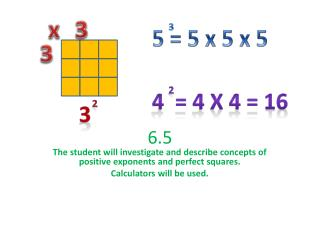DownloadDownload Presentation6.5

# 6.5

Download Presentation## 6.5

- - - - - - - - - - - - - - - - - - - - - - - - - - - E N D - - - - - - - - - - - - - - - - - - - - - - - - - - -
##### Presentation Transcript

1. x 3 3 5 = 5 x 5 x 5 3 6.5 2 4 = 4 x 4 = 16 2 3 The student will investigate and describe concepts of positive exponents and perfect squares. Calculators will be used.

2. Pg EXPONENTS- • Any real number other than zero raised to the zero power is 1. Ex-50=1 100=12370=1 Perfect Square Practice- On graph paper draw and count. Use this button on your calculator 2² 3² 4² 5² 6² Square Root Calculator-use this button on the calculator Find the product (base Yxpower) 85 49 20 20 106 78 80 =______ Which number is the base in 74 ? ____Power?___ 6.5 Vocabulary pg Exponent- A numerical value composed of a power and a base. EX- 53 means 5x5x5= 125 Exponential Notation- In exponential notation, the base is the number that is multiplied, and the exponent represents the number of times the base is used as a factor. EX- 53 5 is the base and 3 is the number of times you multiply 5 times itself. To use your calculator to find any exponential number simply punch in the base, then the Y2 key and then the power. Ex: 24 you would punch in “2 “ then “Y2 “ then “4” and it will equal 2 x 2 x 2 x 2  which is 16. Power- The power, written as a superscript number, symbolizes how many times the base number must be multiplied to find the numerical value of the exponent. EX- 53 3 is the power. Base- The base is the factor that will be multiplied in an exponent. The power tells how many times the base will be multiplied to find the numerical value of the exponent. EX- 53 5 is the base. Power of Ten- A power of ten is an exponent composed of the number ten (10) raised to a power. The power tells how many zeroes will be in the standard form of the exponent. EX- 10³ will have three zeroes in the answer, making it 1,000. If 10³ was written as a product of its factors, it would read 10×10×10=1,000. HINT- just count the zeros, that is your power! Square root- A square root of a number is a number which, when multiplied by itself, produces the given number. EX- the square root of 49 is 7 since 7  7 = 49. Squared- A number multiplied by itself; symbolized by a superscript 2 written above and to the right of a number. EX- 5 squared (5²) would be solved by multiplying 5×5=25. Perfect square- Perfect squares are the numbers that result from multiplying any whole number by itself Ex- 36 = 6  6.   SAME THING AS SQUARED. 62 Cubed- A number, multiplied by itself and then multiplied again by itself. It is symbolized by a superscript 3 written above and to the right of a number. EX- 4 cubed (4³) would be solved by multiplying 4×4×4=64. 43 x2 x2

3. To calculate exponents punch in base, then key, then power base Y xpower To calculate square roots, punch in number and hit this key